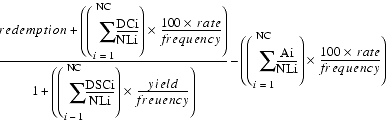# ODDLPRICE

Description Computes the price of a security purchased during a last coupon period that is shorter or longer than the other coupon periods. In addition to the dates, you must specify the rate, redemption value, and yield expected.

Syntax ODDLPRICE ( settlement, maturity, last_coupon, rate, yield, redemption, frequency [, calendar_type] )
Argument Description
settlement The date when the security is traded to the buyer. It must be after last_coupon. Decimal values will be truncated to integers.
maturity The date the security expires and the remaining amount is paid to the investor. Decimal values will be truncated to integers.
last_coupon The date of the last interest payment.
rate The security's annual coupon rate. The coupons pay at this rate divided by frequency.
yield The annual income produced by the security.
redemption The security's redemption value per \$100 face value. This is the amount paid at the settlement date that is not part of any final coupon payment.
frequency The number of interest payments per year. See The frequency Argument for more information.
[calendar_type] Optional. One of five methods of counting days for computing interest. See The calendar_type Argument for more information.

Remarks This function should be used only when the security's last coupon date is a different length than the other coupon dates AND the security was purchased during this odd coupon period.

If all coupon periods are of equal length, use PRICE. If the first coupon period is shorter or longer than the rest, use ODDFPRICE.

Equation...where the codes correspond to values you can compute using the coupon functions, as shown in the following table.

Note The software divides the odd last period into non-paying coupon periods in order to correctly calculate the price. These non-paying periods are referred to as pseudo-periods. The variable i represents the pseudo-periods.
Code Meaning Function
Ai Number of days from last_coupon to settlement. COUPDAYBS
DCi Number of days in each coupon period. COUPDAYS
DSCi Number of days from settlement to the next pseudo-period or to maturity. COUPDAYSNC
NC Number of pseudo-periods. (Fractional values are raised to integers.) COUPNUM
NLi Number of days in each pseudo-period. COUPDAYS

Examples This function returns 1.416517:

`ODDLPRICE("4/19/97","11/25/97","4/1/97",0.05,99.8,100,4)`